## Info

Publication number
JP4574471B2
JP4574471B2 JP2005198591A JP2005198591A JP4574471B2 JP 4574471 B2 JP4574471 B2 JP 4574471B2 JP 2005198591 A JP2005198591 A JP 2005198591A JP 2005198591 A JP2005198591 A JP 2005198591A JP 4574471 B2 JP4574471 B2 JP 4574471B2
Authority
JP
Japan
Prior art keywords
signal
phase
dc offset
complex
points
Prior art date
Legal status (The legal status is an assumption and is not a legal conclusion. Google has not performed a legal analysis and makes no representation as to the accuracy of the status listed.)
Active
Application number
JP2005198591A
Other languages
Japanese (ja)
Other versions
JP2006115463A (en
Inventor

Original Assignee

Priority date (The priority date is an assumption and is not a legal conclusion. Google has not performed a legal analysis and makes no representation as to the accuracy of the date listed.)
Filing date
Publication date
Priority to JP2004271249 priority Critical
Application filed by 株式会社日立国際電気 filed Critical 株式会社日立国際電気
Priority to JP2005198591A priority patent/JP4574471B2/en
Publication of JP2006115463A publication Critical patent/JP2006115463A/en
Application granted granted Critical
Publication of JP4574471B2 publication Critical patent/JP4574471B2/en
Application status is Active legal-status Critical
Anticipated expiration legal-status Critical

## Images

•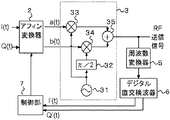•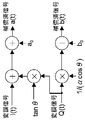•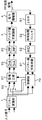•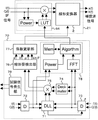•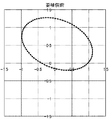•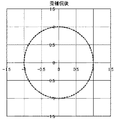•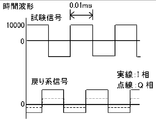•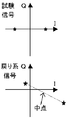•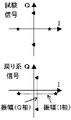•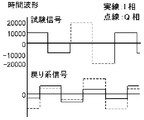•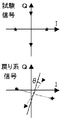•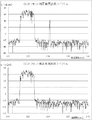•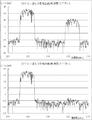•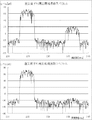•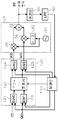•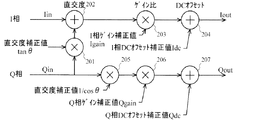•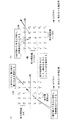•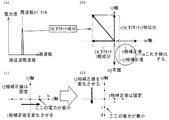•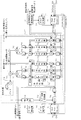## Classifications

• HELECTRICITY
• H03BASIC ELECTRONIC CIRCUITRY
• H03CMODULATION
• H03C3/00Angle modulation
• H03C3/38Angle modulation by converting amplitude modulation to angle modulation
• H03C3/40Angle modulation by converting amplitude modulation to angle modulation using two signal paths the outputs of which have a predetermined phase difference and at least one output being amplitude-modulated
• H03C3/406Angle modulation by converting amplitude modulation to angle modulation using two signal paths the outputs of which have a predetermined phase difference and at least one output being amplitude-modulated using a feedback loop containing mixers or demodulators
• HELECTRICITY
• H04ELECTRIC COMMUNICATION TECHNIQUE
• H04LTRANSMISSION OF DIGITAL INFORMATION, e.g. TELEGRAPHIC COMMUNICATION
• H04L27/00Modulated-carrier systems
• H04L27/32Carrier systems characterised by combinations of two or more of the types covered by groups H04L27/02, H04L27/10, H04L27/18 or H04L27/26
• H04L27/34Amplitude- and phase-modulated carrier systems, e.g. quadrature-amplitude modulated carrier systems
• H04L27/36Modulator circuits; Transmitter circuits
• H04L27/362Modulation using more than one carrier, e.g. with quadrature carriers, separately amplitude modulated
• H04L27/364Arrangements for overcoming imperfections in the modulator, e.g. quadrature error or unbalanced I and Q levels

## Description

The present invention relates to a distortion-compensating quadrature modulator and a radio transmitter, and more particularly to a distortion-compensating quadrature modulator and a radio transmitter for outputting a highly accurate quadrature modulated wave with an analog quadrature modulator.

In a digital modulation method used in a mobile communication system such as W-CDMA or PDC in which a use band of a radio frequency is limited, a highly accurate modulator is required as compared with an analog modulation method such as AM or FM. When a quadrature modulator is configured with a digital circuit, there is no problem, but when configured with an analog element, it is important to compensate for the following three linear distortions.

First, the output signals I (t) and Q (t) of the D / A converter used for the input of the quadrature modulator are completely adjusted for the offset of the DC component generated with respect to the original zero balance modulation signal. Difficult to do. Further, a shift occurs due to a temperature change and a secular change, and a carrier leak component is superimposed on the modulated wave due to the shift of the DC offset.
Second, the average amplitude ratio of the analog modulation signals I (t) and Q (t) is deviated from the original 1, and distortion components are superimposed on the image frequency region.
Third, it is difficult to accurately manufacture a π / 2 phase shifter necessary for a quadrature modulator, and the deviation of the orthogonality causes distortion components to be superimposed on the image frequency region.
In particular, in the direct conversion method in which the circuit scale is expected to be reduced by directly converting the baseband signal to the RF frequency, it is becoming more and more important to solve the problem caused by the analog element.

Conventionally, Non-Patent Document 1 is known as a solution to this problem. The schematic configuration is shown in FIG.
In the figure, 120 is a distortion compensation circuit, 3 is a quadrature modulator, 150 is a detector that detects an RF transmission signal, 160 is an LPF that averages the detection output, and 170 is a control circuit that generates a distortion compensation coefficient and a test pattern. . The affine transformer 121 is configured as shown in FIG. 2, and functions to correct DC offset, IQ gain difference, and orthogonality deviation inherent in the quadrature modulator by giving a0, b0, α, and θ. To do. As a specific control method, a test pattern signal is output with SW2 and SW3 as the controller side, and the output of the LPF 160 is observed to first determine and set the DC offsets a ′ and b ′. Next, SW2 and SW3 Is the affine converter 121 side, SW0 and SW1 are switched to the control circuit side, and α and θ are obtained by observing the LPF160 output level while sending out the test pattern signal.

Hiroshi Suzuki, 1 other, "Affine Transform Linear Distortion Compensation-Application to Linear Signal Transmission including Equalization in Mobile Radio Communication", IEICE Transactions B-II, IEICE, 1992 January, Vol. J75-B-II, No. 1, p. 1-9

As described above, in the prior art, assuming the factory setting or resetting by temporarily suspending operation, each distortion compensation coefficient is derived by sending a test pattern signal. Each distortion compensation coefficient cannot be derived during transmission of the modulation signals I (t) and Q (t). Therefore, the conventional technology cannot be applied to applications that cannot secure a transmission pause time for deriving each distortion compensation coefficient during operation, such as a base station that performs transmission at random timing, and cannot follow temperature changes and aging changes. There was a problem.
Alternatively, it is possible to improve the prior art and switch the modulated signal to another quadrature modulator with a switch to secure the modulation output while sending out the test pattern signal, but complete non-stop switching is still difficult. Yes, it is not realistic in terms of cost and follow-up performance.

The present invention has been made from the above-described background, and an object thereof is to provide a distortion-compensating quadrature modulator that can optimally update each distortion-compensating coefficient even during operation.
It is another object of the present invention to provide a compensator that can efficiently compensate for a DC offset generated in a complex amplitude signal to be processed.

The input complex amplitude signal (modulating signal) I (t) and Q (t) is affine transformed based on the affine transformation coefficient, and the compensated signals a (t) and b (t) are output. Means,
A quadrature modulator that quadrature modulates a local oscillation signal based on the input compensated signal and outputs a modulated signal that is a real signal;
A quadrature that outputs a complex feedback signal I ′ (t) and Q ′ (t) by removing a carrier wave component from the input modulated wave signal or a signal obtained by performing frequency conversion or amplification on the modulated wave signal. Detection means;
Linear distortion remaining in the complex feedback signals I ′ (t) and Q ′ (t) is extracted as a distortion coefficient, and the current affine transformation coefficient is updated to the new affine transformation coefficient in accordance with an update formula including the distortion coefficient. And control means for resetting the affine transformation means,

Test signal generating means for generating a complex test signal having a plurality of points on the IQ plane as signal points;
Affine transformation means for affine transformation of the input complex test signal based on a given affine transformation coefficient and outputting compensated signals a (t) and b (t);
A quadrature modulator that quadrature modulates a local oscillation signal based on the input compensated signal and outputs a modulated wave signal that is a real signal;
A quadrature detection means for removing a carrier wave component from the input modulated wave signal or a real signal based on the modulated wave signal and outputting a complex feedback signal;
Linear distortion detection means for extracting linear distortion remaining in the complex feedback signal as four distortion coefficients respectively corresponding to an I-phase component of a DC offset, a Q-phase component of a DC offset, an IQ gain ratio, and an orthogonality shift; A distortion compensated quadrature modulator comprising:
The linear distortion detection means calculates the distance between the midpoint of the two points on the IQ plane of the corresponding complex feedback signal and the origin with respect to two points symmetrical about the origin on the IQ plane of the test signal. Determining and determining an I-phase component and a Q-phase component of the DC offset based at least on a distance between the midpoint and the origin;
With respect to four points, which are a set of two points arranged symmetrically with respect to the origin on the IQ plane of the test signal at an interval of 90 degrees, 2 of each set on the IQ plane of the corresponding complex feedback signal A process for determining a distance between the points, and determining the IQ gain ratio based on the distance between the two points in each set;
With respect to four points, which are a set of two points arranged symmetrically with respect to the origin on the IQ plane of the test signal at an interval of 90 degrees, 2 of each set on the IQ plane of the corresponding complex feedback signal A distortion-compensating quadrature modulator, comprising: obtaining a value corresponding to a deviation from a right angle of an angle at which the connecting lines of points intersect each other, and determining the orthogonality deviation.

In a transmitter that transmits multiple communication channels,
Based on the complex baseband signals for N (N is an integer of 1 or more) carriers that have been input, digital modulation is performed at a frequency corresponding to each carrier to synthesize them as complex IF signals I (t) and Q (t). An output digital modulation circuit;
A (t) = I (t) + Q (t) · tan θ + a where a, b, tan θ, 1 / (α cos θ) are affine transformation coefficients to the input complex amplitude signals I (t) and Q (t).
b (t) = Q (t) / (αcos θ) + b
An affine transformation circuit that outputs compensated signals a (t) and b (t) compensated for DC offset, IQ gain ratio, and orthogonality deviation;
A D / A converter for converting the input compensated signals a (t) and b (t) from digital to analog and outputting the converted signals,
A quadrature modulator that quadrature modulates a carrier wave signal based on the input D / A converter output and outputs an RF signal;
A power amplifier that amplifies and outputs an input RF signal; and
A quadrature detection circuit that digitally quadrature-detects a signal based on the input power amplifier and outputs complex feedback signals I ′ (t) and Q ′ (t) having an IF frequency substantially equal to that of the digital modulation unit;
The complex IF signal and the complex feedback signal that have been input are subjected to complex conjugate multiplication, and the deviation angle is detected as a phase difference φ between the complex IF signal and the complex feedback signal, and phase rotation is performed from the complex feedback signal. A phase rotation removal circuit for removing and outputting rotation removal signals I r (t) and Q r (t);
The linear distortion remaining in the complex feedback signals I ′ (t) and Q ′ (t) is converted into four distortion coefficients a corresponding to the I-phase component and Q-phase component of the DC offset, IQ gain ratio, and orthogonality deviation, respectively. Linear distortion detecting means for extracting ', b', α, sin θ,
Coefficient update means for updating the current affine transformation coefficient to the new affine transformation coefficient and resetting it in the affine transformation means according to an update formula including the distortion coefficient, and a wireless transmitter comprising:
When detecting the linear distortion when the wireless transmitter is not in operation, the linear distortion detecting means is a signal point that is point-symmetric about the origin of the test signal composed of a rectangular wave on the I phase or the Q phase. For the two points, find the midpoint on the IQ plane of the corresponding complex feedback signal, set the distance between the midpoint and the origin as the magnitude of the DC offset, and set the DC offset with the phase changed in the affine transformation means While detecting the midpoint and determining the I-phase component and Q-phase component of the DC offset from the magnitude and phase of the DC offset that minimizes the distance between the midpoint and the origin, and time-division For a set of two point-symmetric signal points around the origin of the test signal consisting of a rectangular wave on the I phase and a rectangular wave on the Q phase, each set of 2 on the IQ plane of the corresponding complex feedback signal. Find the distance between each point, A process of determining the IQ gain ratio based on the distance between the two points of each set, and a test signal comprising a rectangular wave on the I phase and a rectangular wave on the Q phase that are time-divided at intervals of one period Corresponds to a deviation from the right angle of the angle at which the connecting lines of the two points of each set on the IQ plane of the corresponding complex feedback signal intersect with the set of two point-symmetric signal points around the origin of Obtaining a value, and determining the orthogonality deviation,
When the wireless transmitter detects linear distortion during operation, the linear distortion detection means determines the four distortion coefficients as a ′ = <I r (t)>.
b ′ = <Q r (t)>
α ′ = (<I r (t) 2 > / <Q r (t) 2 >) 1/2
sin θ ′ = − <I r (t) Q r (t)> / {<I r (t) 2 ><Q r (t) 2 >} 1/2
(Where <> means an average value for a long period of time), and the coefficient updating means uses the update equation as a n = a n-1 −μa ′.
b n = b n−1 −μb ′
α n = α n−1 × (α ′) 1 / m
sin θ n = sin θ n−1 + μsin θ ′
cos θ n = (1-sin 2 θ n ) 1/2
tan θ n = sin θ n / cos θ n
A wireless transmitter characterized by using the above.
Further, the long-term average is a time average equivalent to 4096 chips or more.
The wireless transmitter further includes a delay lock loop that matches and delays the complex IF signal and the complex feedback signal with a time accuracy less than the reciprocal of a sample rate of the complex IF signal, Is a delay time difference given to the late path and the early path, which is less than the reciprocal of twice the bandwidth of the complex IF signal and more than twice the variable step unit of the delay time of the FDF. .

In a compensator for compensating for a DC offset of an input complex amplitude signal,
DC offset correction means for giving an I-phase correction value and a Q-phase correction value of DC offset to the I-phase component and Q-phase component of the input complex amplitude signal, respectively.
Level detection means for detecting a level of the feedback signal by feeding back the complex amplitude signal to which the correction value is given by the DC offset correction means;
Correction value control means for controlling the I-phase correction value and the Q-phase correction value given by the DC offset correction means based on the level detected by the level detection means,
The correction value control means initially sets the I-phase correction value and the Q-phase correction value to the I-phase component value and the Q-phase component value at each point among a plurality of points provided at predetermined intervals on the IQ plane. When the value is set in the DC offset correction means as a value, a point where the level detected by the level detection means becomes minimum when the level of the input complex amplitude signal is 0 is searched. The searched point is set as the current center point and the predetermined interval is made smaller this time than the previous time, the search is performed at least once, and the I-phase component value and Q of the finally searched point are A phase component value is adopted as the I-phase correction value and the Q-phase correction value and set in the DC offset correction means.
Compensator characterized by that.

According to the distortion-compensating quadrature modulator and the radio transmitter according to the present invention, the distortion compensation coefficient (affine transformation coefficient) is always updated optimally even during operation (I (t), Q (t) transmission). Can cope with temperature change and aging.
Further, according to the compensator according to the present invention, it is possible to efficiently compensate for the DC offset generated in the complex amplitude signal.

FIG. 1 is a configuration diagram of a constantly updating distortion compensating quadrature modulator according to an embodiment of the present invention.
2 is an affine converter, 3 is a quadrature modulator, 5 is a frequency converter that converts an RF frequency to an intermediate frequency band, and 6 is an analog intermediate frequency signal that is digitized to generate quadrature detection signals I ′ (t), Q ′ ( It is a digital quadrature detector that converts to t). The affine converter 2 has the same configuration as that shown in FIG. The digital quadrature detector 6 is premised on the use of a digital quadrature detector because the DC offset, IQ gain ratio, and IQ quadrature distortion of the quadrature detector do not occur in principle. A control unit 7 updates each distortion compensation coefficient used in the affine converter.

Hereinafter, an algorithm for updating each distortion compensation coefficient in the control unit 7 will be described.
In principle, the correction error is extracted from the digital quadrature detection output I ′ (t) and Q ′ (t) signals, which are outputs based on the affine transformation coefficients set in the affine transformer 2 at a certain time, and updated. It takes the formula and pulls it to the optimum value.
Initial state of n times, the coefficient update by performing the DC offset of the I (t) side determined a n, Q (t) side of the DC offset to b n, the IQ gain ratio alpha n, IQ orthogonality deviation The sine of the angle is set as sin θ n , the DC offset on the I (t) side remaining in the current digital quadrature detection output is a ′, the DC offset on the Q (t) side is b ′, and the IQ gain ratio is When the sine of α ′ and IQ orthogonality deviation angle is set as sin′θ, the following calculation is performed using the digital quadrature detection outputs I ′ (t) and Q ′ (t).

First, the phase difference φ between the modulation signal (I (t), Q (t)) and the corresponding digital quadrature detection signal (I ′ (t), Q ′ (t)) is obtained, and phase rotation is performed. To compensate.
φ = Arg [(I (t) + jQ (t)) (I ′ (t) −jQ ′ (t))] [Expression 1]
I r (t) = I ′ (t) cos φ−Q ′ (t) sin φ (Formula 2)
Q r (t) = Q ′ (t) cos φ + I ′ (t) sin φ (Formula 3)
Here, Arg [] represents a complex argument. Note that the delays in the affine converter 2, the quadrature modulator 3, and the frequency converter 5 are not particularly considered in the above <Expression 1> to <Expression 3>.

Next, a strain coefficient indicating each residual strain is obtained by the following equation.
a ′ = <I r (t)> (Formula 4)
b ′ = <Q r (t)> (Formula 5)
α ′ = (<I r (t) 2 > / <Q r (t) 2 >) 1/2 (Formula 6)
sin θ ′ = − <I r (t) Q r (t)> / {<I r (t) 2 ><Q r (t) 2 >} 1/2 (Expression 7)
Here, <> represents a long-term average value.

Next, the affine transformation coefficient is updated using the distortion coefficient obtained by <Expression 4> to <Expression 7>.
a n = a n−1 −μa ′ (Formula 8)
b n = b n−1 −μb ′ (formula 9)
α n = α n−1 × (α ′) 1 / m (Formula 10)
sin θ n = sin θ n−1 + μsin θ ′ (Formula 11)
(If θ≈0, it can be assumed that sinθ≈θ, so approximation can be used.)
cos θ n = (1-sin 2 θ n ) 1/2 (Formula 12)
tan θ n = sin θ n / cos θ n (Formula 13)
However, μ and m are step parameters, usually μ is about 1/4 to 1/512, and m is an integer of 1 or more.

In this embodiment, the present invention is applied to a wireless transmitter (wireless base station) that transmits a 4-carrier W-CDMA (Wide-Code Division Multiple Access) signal. In the present embodiment, an algorithm for updating the distortion compensation coefficient using a special test signal is further provided in order to speed up the adjustment process when not in operation such as at the time of factory shipment. In addition, a present Example is only an example of this invention, This invention is not limited to the numerical value, the processing order, and implementation means (hardware) which were specifically described in description of this Example, It may be different.

FIG. 3 is a configuration diagram of the radio transmitter according to the present embodiment, and shows only a transmission system after baseband processing and a return system associated therewith.
The digital modulation unit 1 performs band limitation, digital orthogonal modulation, and the like on the input baseband signal (IQ signal) of each carrier, and then synthesizes and outputs the result. The output of the digital modulator 1 is an IF signal in the IF (Intermediate Frequency) band.
The distortion correction unit 21 performs DPD (Digital PreDistortion) processing on the output of the digital modulation unit 1 in addition to correcting DC offset, IQ gain ratio, and orthogonality deviation using affine transformation.
The D / A converter 22 converts each of the I phase and Q phase of the digital signal input from the distortion correction unit 21 into an analog signal.
The LPF 23 removes frequency components other than the target IF frequency band from the output of the D / A converter 22.
The analog quadrature modulator 3 performs analog quadrature modulation using the output of the LPF 23, and outputs a modulated wave signal (real signal) in a target RF (Radio Frequency) band.
The power amplifier 4 amplifies the output of the analog quadrature modulator 3 to the power necessary for wireless transmission.
When a part of the output of the power amplifier 4 is input, the mixer 51 down-converts to the IF frequency.
The band limiting filter 52 performs band limitation on the output of the mixer 51 to remove frequency components other than the target IF frequency band.
The A / D converter 62 converts the analog signal input from the band limiting filter 52 into a digital signal.
The digital quadrature detector 61 digitally quadrature-detects the output of the A / D converter 62 and outputs an IQ signal having an IF frequency equal to the output of the digital modulation unit 1.
The controller 70 detects the DC offset, IQ gain ratio, and orthogonality deviation, updates the four distortion coefficients a ′, b ′, α ′, and sin θ ′ and outputs them. These detections are performed based on the output of the digital modulation unit 1 and the output of the digital quadrature detector 61 during operation, and are specially provided by the control unit 70 to the distortion correction unit 21 during non-operation (factory adjustment, etc.). This is performed based on the test signal and the output of the digital quadrature detector 61.
The switch 63 connects the input of the distortion correction unit 21 to the output of the digital modulation unit 1 during operation, and connects to the test signal output from the control unit 70 during factory adjustment.

Next, the detailed configuration of each part will be described.
A digital complex baseband signal (IQ signal) having a sampling rate of a chip rate (3.84 MHz) is input to the digital modulator 1 for four carriers. Each digital complex baseband signal is a composite signal of multiple channels multiplexed with a spreading code, and each channel is power controlled and has a different amplitude, so each digital complex baseband signal has an arbitrary value on the IQ plane. Can take. Each digital complex baseband signal is first subjected to filtering of the root roll-off characteristic for each of the I and Q components by four FIR filters, and the sampling frequency is up to 92.16 MHz (24 times of 3.84 MHz). As it is oversampled, the image components are removed. Next, the output (IQ signal) of the FIR filter of each carrier is subjected to complex multiplication with a complex local signal represented by (cos (2πf i ), sin (2πf i )), (i = 1..4). The Since complex multiplication is performed, no new image is generated by this, and the frequency is simply converted to the IF band. f1 to f4 are, for example, 15.36, 20.36, 25.36, 30.36 MHz. Next, the complex multiplication output (IQ signal) of each carrier is synthesized additively. Finally, the combined output is subjected to peak suppression processing by a limiter (window function method) that suppresses a signal in the vicinity of the peak with a weight of the window function, and becomes an output of the digital modulation unit 1.

The distortion correction unit 21 has a configuration in which the DPD unit 64 and the affine transducer 2 are connected in series. The DPD unit 64 calculates the instantaneous power of the input IF signal, reads the distortion corresponding to the power from the distortion compensation table (LUT), and multiplies the input IF signal. The distortion compensation table stores reverse characteristics of nonlinear distortion generated in the power amplifier 4 or the like. The affine converter 2 has the same configuration as that of the prior art and is as shown in FIG. The distortion compensation table of the DPD unit 64 and the four affine converter coefficients a, b, tan θ, 1 / (α cos θ) of the affine converter 2 are updated by the control unit 70 so as to reduce the distortion. Input / output of the DPD unit 64 and the affine converter 2 is performed in real time, but the distortion compensation table and the coefficient may be updated by batch processing.

The analog quadrature modulator 3 is the same as the analog quadrature modulator 3 of FIG. In FIG. 1, a local oscillator 31 generates an RF band sine wave as a carrier. The phase shifter 32 shifts the phase of the output of the local oscillator 31 by π / 2. Multipliers 33 and 34 multiply the LPF 23 output I-phase and Q-phase by the local oscillator 31 output and the phase shifter 32 output, respectively. The adder 35 synthesizes the outputs of the multipliers 33 and 34 in an additive manner. Finally, the BPF (not shown) passes the output of the adder 35 through only the transmission band of about 1.95 GHz and becomes the output of the analog quadrature modulator 3. The analog quadrature modulator 3 is realized by, for example, an MMIC (Microwave Monolithic Integrated Circuit). A high C / N (Carrier-to-Noise Ratio) can be obtained by a method in which the I and Q phases are individually D / A converted and analog quadrature modulated as in this embodiment.

When a part of the output of the power amplifier 4 taken out by the coupler is input, the mixer 51 multiplies the local signal of the local oscillator (not shown) and down-converts it to the IF frequency. The local oscillator here is not necessarily the same as the local oscillator 31 in the analog quadrature modulator 3. In this embodiment, the return system from the mixer 51 to the control unit 70 is also used as a DPD, and therefore requires at least the same bandwidth as the transmission system. For convenience of performing digital quadrature detection, the A / D converter 62 has 2 Double or more bandwidth is required. Therefore, the return IF is higher than the transmission IF.

The band limiting filter 52 performs band limitation on the output of the mixer 51 to remove frequency components other than the target IF frequency band. The bandwidth of the target IF frequency band is about ½ of the sampling frequency of the A / D converter 62, and the center frequency is, for example, (3/4) × 92.16 MHz.

The A / D converter 62 converts the analog signal input from the band limiting filter 52 into a digital signal at a sampling frequency of 2 × 92.16 MHz.

The digital quadrature detector 61 multiplies the output of the A / D converter 62 by digital local signals cos (2πfb) and sin (2πfb) having a relationship of perfect quadrature and equal amplitude, and the multiplication results are respectively I phase and Q Output as a phase. For example, fb is (1/2) × 92.16 MHz. If necessary, the second harmonic image component generated by the digital quadrature detector is deleted and down-sampled to the same sample rate (92.10 MHz) as the IF signal.

The internal configuration of the control unit 70 is shown in FIG.
The buffers 72 and 73 are input from the digital modulation unit 1 (IF signals I (t) and Q (t)) and input from the digital quadrature detector 61 (digital quadrature detection signals I ′ (t) and Q ′ ( Each of t)) is temporarily stored. The buffer 73 functions as variable delay means because the read timing is controlled.
A DLL (Delay Locked Loop) 71 reads signals temporarily stored from the buffers 72 and 73, respectively, and controls the read (or write) timing so that the correlation between the signals is maximized. The sliding correlator (SC) in the DLL 71 performs complex conjugate multiplication represented by <Equation 1> and a weighted average of the multiplication results, and the real part and the imaginary part of the SC output are the SC output. The values divided by the size correspond to cos φ and sin φ in <Expression 2> and <Expression 3>, respectively. When the local oscillation signal based on the common source oscillation is used in the transmission system and the return system, there is almost no fluctuation of φ, so it is not always necessary to update and output cos φ and sin φ for each sample.
The decimator 74 reduces the sample rate to about 2 to 4 times the chip rate (that is, the sample rate of the modulation signal) by thinning out the digital quadrature detection signal read out by the DLL 71. This is because even if there are more sample rates, only the same modulation signal is calculated redundantly, which is statistically meaningless and accuracy is not improved much.
Multiplier 75 performs operations of <Expression 2> and <Expression 3> on the digital quadrature detection signal output from decimator 74 to compensate for phase rotation.
The linear distortion detector 76 performs the calculations of <Expression 4> to <Expression 7> using the output of the multiplier 75, and outputs a ′, b ′, α ′, and sin θ ′ for each time averaged over a long period. According to the simulation, in order to suppress the linear distortion by 60 dBc or more, it is necessary to perform an average for a long period of about 5000 chips (4096 chips or more is one standard, and the number of samples is about 2 to 4 times the number). Compared with the weighted average for the long-term average, it is better in terms of convergence to add all the samples after the last update of the affine transformation coefficient and divide by the number of samples at the end.
The coefficient updating unit 77 performs the operations of <Expression 8> to <Expression 13>, updates the four affine transformation coefficients, and outputs them. <Expression 11> can also be interpreted as the following <Expression 11 ′>. The first approximation means Taylor expansion, and the second approximation is because sin θ≈θ and cos θ n are approximated to 1 and incorporated into μ.
sin θ n + 1 = sin (θ n + μθ ′)
≒ sinθ n + cosθ n・ μθ '
≈sin θ n + μ sin θ ′ (Formula 11 ′)
In addition, approximation can be appropriately used as long as an appropriate approximation is given in θ → 0 and α → 1. In this embodiment, the four affine transformation coefficients a, b, tan θ, 1 / (αcos θ) are distortion coefficients a ′, b ′, α ′, sin θ ′ representing residual distortion that cannot be compensated by the affine transformer 2. The parameters a n , b n , α n , and sin θ n indicating the inverse characteristics of the distortion of the quadrature modulator 3 (or the distortion itself) are first updated so as to approach the true values. The affine transformation coefficient is uniquely determined from the parameters. That is, the affine transformation coefficient is updated via the parameter.
The DPD control unit 78 receives the transmission system signal and the return system signal whose delays are equalized by the DLL 71, and performs adaptive control to update the LUT so that the distortion component included in the return system signal is minimized. Although FIG. 4 illustrates an example of performing FFT (Fast Fourier Transform) on the return signal and evaluating the out-of-band power as a distortion component, the present invention is not limited to this, and the difference between the transmission signal and the return signal is expressed as an error vector. May be detected.
The test signal generator 79 generates a test signal used for distortion detection during non-operation. The details of the test signal will be described later, but it may be given to the digital modulation unit 1 as a baseband signal or to the distortion correction unit 2 as an IF signal.

An example in which distortion compensation is performed by the update formulas of <Formula 8> to <Formula 13> is shown. FIG. 5 shows a case where I (t) = cos ωt and Q (t) = sin ωt are transmitted in a strained state (distortion amount: a ′ = 0.3, b ′ = 0.7, α = 0.8, θ = −π / 8), FIG. 6 shows a simulation result when the distortion compensation of the present proposal is performed. It was confirmed that if C = 1/64 and m = 2 were sequentially used as the coefficients, C / N = 60 dB or more could be finally obtained.

Next, a distortion compensation coefficient update algorithm using a special signal, which is a feature of the present embodiment, will be described.
In general, a delay, a level difference, and a phase difference are generated between the transmission system signal and the return system signal shown in FIG. 3, and these values are unknown because they include analog circuits. The delay and level difference can be detected and corrected independently, but the phase difference cannot be detected correctly if there is a DC offset, IQ gain ratio, or orthogonality deviation. Conversely, DC offset, IQ gain ratio, and orthogonality deviation cannot be detected correctly if there is a phase difference. For this reason, in the distortion-compensated quadrature modulator described in [Best Mode for Carrying Out the Invention], after obtaining the phase difference φ, the DC offset a, b, the IQ gain ratio α, the orthogonality deviation θ, and the phase difference φ. The four error items are corrected little by little and set to the optimum value. However, in the method in which the four error items are gradually driven to the optimum value together, when these errors start to be unknown, the detection time of each error (detection value convergence time) becomes long.
Therefore, in this embodiment, distortion detection is performed more directly using a rectangular wave test signal. A distortion detection method using a rectangular wave signal will be described in three parts: (i) DC offset, (ii) IQ gain ratio, and (iii) orthogonality deviation.

(i) DC offset detection method First, a test signal as shown in FIG. 7 is transmitted as an input signal. Here, the amplitude and period of the rectangular wave may be optimal for the transmission amplifier apparatus system. The return signal subjected to quadrature detection is a rectangular wave signal whose phase is shifted from the transmission signal. Here, as shown in FIG. 8, two transmission signals are transmitted at symmetrical positions across the origin on the I axis (or no problem with the Q axis) on the IQ plane. These two points come to positions (coordinates) where the DC offset is superimposed and the phase is shifted on the return system side. Therefore, the magnitude of the DC offset can be determined by obtaining the midpoint between the two return system signals. Since the magnitude of the DC offset has been found, a vector having this magnitude is set to an arbitrary phase, the same rectangular wave signal is transmitted again, and the midpoint of the two return system signals is closest to the origin Becomes the phase of the DC offset. Accordingly, the DC offset values of the I phase and the Q phase are determined from the magnitude and phase of the detected DC offset. The midpoint may be obtained by appropriately determining the two points as a symbol and calculating the average of the two points, but it may be an average time (for example, a multiple of a rectangular wave period) at which the appearance probabilities of the two points are equal. For example, it may be a simple average value of all measured samples. Further, by measuring the delay time of the rising edge of the rectangular wave of the test signal and the return system signal, an approximate value of the delay can be obtained and given to the DLL 71 as an initial value.

FIG. 12 shows frequency spectrum diagrams before and after correcting the DC offset. This spectrum diagram is a frequency spectrum diagram of an analog quadrature modulator output signal obtained by computer simulation. The signal is one carrier of W-CDMA (carrier frequency 224.64 MHz). It can be seen that the DC component that appears at the center frequency of 230.4 MHz in the spectrum before correction (appears due to the presence of a DC offset) drops to a level near the noise floor after correction.

(ii) IQ gain ratio detection method As the input signal, the same test signal as that at the time of detecting the DC offset as shown in FIG. First, as shown in FIG. 9, when a rectangular wave signal is transmitted only for the I phase, the distance between two points on the IQ plane detected by the return system is detected as the I phase gain. Similarly, the distance between two points on the IQ plane detected by the return system when a rectangular wave signal is transmitted only in the Q phase is detected as the Q phase gain. The IQ gain ratio is detected by obtaining the ratio between the I phase gain and the Q phase gain.
FIG. 13 shows frequency spectrum diagrams before and after correcting the IQ gain ratio. This spectrum diagram is obtained by the same computer simulation as in FIG. It can be seen that the component (appearing by the IQ gain ratio) that appears as the frequency (236.16 MHz) on the opposite side of the center frequency 230.4 MHz in the spectrum before correction falls to a level near the noise floor after correction.

(iii) Detection Method of Orthogonality Deviation A rectangular wave signal in which the I and Q phases as shown in FIG. 10 are alternately excited with different amplitudes every cycle is transmitted as an input signal. In the return system, four points are detected on the IQ plane as shown in FIG. Since the orthogonality between the line connecting the two points corresponding to the I-phase side and the line connecting the two points corresponding to the Q-phase side is shifted (I phase and Q phase can be distinguished by the difference in amplitude value), 4 The orthogonality deviation θ is detected from the coordinates of the points.
Specifically, if two-point difference vectors are obtained for both I and Q phases, and the inner product of the difference vectors is normalized (with a magnitude of 1), cos (π / 2−θ) = sin θ is obtained. There is no practical problem if the I and Q phases are distinguished by the distance from the origin of the signal point. However, if sin θ is a value close to 0, the distance from the origin is the distance between the second and third signal points. What is necessary is just to replace I and Q determination. Alternatively, it can be easily distinguished by corresponding to the transmission timing of the test signal.
FIG. 14 shows frequency spectrum diagrams before and after correcting the orthogonality deviation. This spectrum diagram is obtained by the same computer simulation as in FIG. It can be seen that the component (shown by the orthogonality deviation) that appears in the frequency (236.16 MHz) opposite to the center frequency 230.4 MHz in the spectrum before correction falls to a level near the noise floor after correction.

According to this embodiment, it is possible to detect and correct DC offset, IQ gain ratio, and orthogonality deviation in a shorter time than in the past using a rectangular wave signal when not in operation. Further, since the phase difference and the DC offset can be detected independently, the detection accuracy is improved. After correcting these, it is possible to detect and correct the phase difference alone. In addition, during operation, the affine transformation coefficient is always maintained at the value that seems to be optimal at that time, so compared to the method of perturbing the affine transformation coefficient and detecting changes in residual distortion with a lock-in amplifier, etc. It is preferable for application to a mobile communication base station apparatus in which distortion is small and signal quality is strictly defined.

This embodiment will explain that distortion can be corrected by affine transformation other than that described in [Best Mode for Carrying Out the Invention]. The affine transformation performed by the affine transformer of FIG. 2 and the linear distortion generated by the analog quadrature modulator are generally expressed by the following <Expression 14> and <Expression 15>.

An object of the present invention is to obtain a compensation matrix L = [l ij ] that compensates for the linear distortion of the analog quadrature modulator represented by <Equation 15>, that is, DC offset, IQ gain ratio, and orthogonality deviation. The DC offsets c and d can be removed by a ′ and b ′ obtained by linearly converting c and d by L and multiplying by −1, and the distortion compensation matrix L is not affected even if the DC offset changes. Therefore, if ignored for the sake of simplicity, it can be said that the object of the present invention is to obtain a matrix L in which the matrix product ML is an orthogonal matrix (orthogonal transformation) for the distortion matrix M = [m ij ]. If L = M −1 is obtained, ML = E (unit matrix) becomes an orthogonal matrix. Considering a matrix (corresponding to depriving the size of ML and the degree of freedom of rotation) having an element of 1 or 0 in any 1 row or 1 column as M, for example,

Then, l 12 = −m 21 m 22 and l 22 = 1 / m 22 . Therefore, it can be seen that the linear transformation of FIG. 2 was an inverse transformation of linear distortion given by m 12 = −α sin θ and m 22 = α cos θ. Also,

ML = E,

L 21 = −m 21 / m 22 and l 22 = 1 / m 22 in ML are also ML = E, and l 21 = tan θ, l 22 = 1 / (α cos θ), m 21 = −α sin θ, and m 22 = α cos θ. It is also possible to replace FIG. 2 with a linear transformation.
There are various types as described above, but there are also differences depending on how to measure θ, such as whether to shift θ from the orthogonal state or to orthogonalize the coordinates shifted by θ. In general, the value of θ differs between the former and the latter measurement methods. For example, in <Equation 17>, even if the sign of θ of M is reversed and the reciprocal of α is taken, it does not match L, but θ → 0 and α → 1. Match.

The compensation matrix L does not have to be an inverse matrix of the distortion matrix M. For M in <Equation 16>, l 11 = 1, l 12 = −m 21 , l 21 = 0, l 22 = (1 + m 21 2 ) / M 22 , or l 11 = {− l 12 (m 22 + m 21 l 12 ) / m 21 } 1/2 , l 12 = −m 21 m 22 / (1 + m 22 2 ), l 21 = 0, ML can be an orthogonal matrix even with L given by l 22 = 1. On the other hand, L in <Equation 16> can make ML orthogonal to an arbitrary distortion matrix M (however, the size is not normalized). Therefore, any type may be adopted as M and L used in the present invention. For example, for a strain M such that m 21 = −α sin θ and m 22 = α cos θ,

It becomes. However, even if an approximation such as 1 / cos θ = 2− (1−sin 2θ) is used, the equation tends to be more complicated than L of the inverse matrix.

Actually, for unknown M, two elements such as <Equation 16> are fixed at 1 and 0, and sin θ is compensated by a matrix L having α as a parameter. If the final L is obtained by iterative calculation as in the best mode, the correction value of L may not be able to accurately form an ML matrix in all θ and α. In other words, anything is acceptable as long as ML is close to an orthogonal matrix as much as possible at the time of convergence (θ → 0, α → 1). Therefore, the difference in how θ is measured is not a problem, and the matrix L should be easy to calculate as in [Best Mode for Carrying Out the Invention].

In principle, the update is performed by multiplying the newly obtained L ′ (correction value) from the left by multiplying the current L (n) from the left to L (n + 1), and the newly obtained linearity of a ′ and b ′. The transformation L −1 (n + 1) is updated to a n + 1 and b n + 1 by adding the current a n and b n .
In [Best Mode for Carrying Out the Invention], the inverse characteristic of the total distortion of the quadrature modulator 3 is used as a parameter, and the true value of the parameter is obtained by iterative calculation and then converted into an affine transformation coefficient uniquely. ing.
As another approximation, for example, when θ = 0 and α = 1, the value is 0, and in the vicinity thereof, a differential coefficient (θ, θ, L (n + 1) = L′ L (n)) is the same as L ′. A simple function f (θ, α) having a partial differential coefficient of α and a magnitude of the derivative smaller than a value obtained by multiplying the above-described derivative of L by the step size μ or the like is represented by the current L (n ) May be added directly. As f (θ, α), any approximation may be used as long as sufficient accuracy for convergence is obtained in the vicinity of θ = 0 and α = 1. As an example, the following update formula can be used instead of <Formula 10> to <Formula 13>.

The initial value L (0) is obtained in the same manner as [Best Mode for Carrying Out the Invention].

In the present embodiment, the detailed configuration of the DLL 71 and its surroundings described in the first embodiment will be described. FIG. 19 is a block diagram of the DLL 71 ′ and its surroundings in this embodiment. The present embodiment is characterized in that a non-integer delay filter (FDF: Fractional Delay Filter) is provided in order to perform highly accurate delay alignment between a transmission system and a return system signal required for detection of linear distortion. And Hereinafter, description will be made with reference to FIG. 19, but unless otherwise specified, this embodiment also has the configuration shown in FIG.
Reference numerals 72 'and 73' denote dual port memories (DPM), which correspond to the buffers 72 and 73 of the first embodiment. The dual port memory has two ports A and B composed of an address bus and a data bus, and can be accessed independently from each port. DPMs 72 ′ and 73 ′ receive IF signals I (t) and Q (t) and digital quadrature detection signals I (t) ′ and Q (t) ′ from port A, respectively, and are shared by address generator 701. Are stored at the same address according to the write address given to each. This operation is always performed.
Address generator 702 provides a read address to port B of DPMs 72 'and 73'. However, the address given to the DPM 73 ′ is offset by the offset assigning unit 703, and adjusting the offset changes the relative delay time of the signals that are primarily stored and read in the DPMs 72 ′ and 73 ′. be able to.
The FDF 705 is composed of independent I-phase and Q-phase FIR (Finite Impulse Response) filters. When a signal read from the DPM 72 'is input, the FDF 705 performs a delay close to the time of the number of samples that is half the number of taps. Output. The delay time is determined by a given tap coefficient, and the delay time is controlled in units of one sample time or less by a known method.
The delay 711 delays the output of the FDF 705 by one sample time (Ts) and outputs it as a transmission system signal to the DLL main body and the DPD control unit 78 (see FIG. 4). The delay 711 may be omitted.
The delay 715 delays the output of the DPM 73 ′ by one sample time (Ts) and outputs it as a return signal to the decimator 74 (see FIG. 4), the DPD control unit 78, and the like.

In the DLL main body, the delay time set in the FDF is adjusted so that the difference between the cross-correlation value powers when the transmission system signal and the return system signal are intentionally shifted by ± Ts becomes zero.
First, delays 712 and 713 delay the output of DPM73 ′ by 2Ts and Ts, respectively, and multipliers 721 to 723 perform complex multiplication of the output of delay 711 and the output of delay 712, DPM73 ′, and delay 713, respectively. And output.
The averaging units 731 to 733 accumulate and add the outputs of the multipliers 72 1 to 723 by a predetermined number of samples (for example, 256), respectively, and the late path, the early path, and the normal path (normal) path) Output as correlation value. The averaging units 731 to 733 and 734 to 736 have the same structure, and only the internal configuration of the 731 is illustrated.
The normalizing unit 741 normalizes the magnitudes of the correlation values output from the averaging units 731 to 733 based on the normal path correlation value output from the averaging unit 733, and outputs the normalized values. As a result, the correlation value is brought close to a value that reflects only the delay time error without depending on the amplitude. The normalized normal path correlation value is output to the multiplier 75 (see FIG. 4) as a compensation amount for the phase rotation φ because the I phase is cos φ and the Q phase is sin φ.
When receiving the late path and early path correlation values normalized by normalization section 741, power generation sections 751 and 752 calculate and output the sum of squares of the I-phase and Q-phase, respectively.
Adder 761 subtracts the early path correlation value power output from power generation section 752 from the late path correlation value power output from power generation section 751 and outputs the result. The output of the adder 761 is positive when the transmission system signal is delayed from the return system signal, and is negative when the transmission system signal is advanced.
A configuration similar to the configuration described above is configured by delays 714 and 715, multipliers 724 to 726, averaging units 734 to 736, normalization unit 744, power generation units 754 and 755, and adder 762, and also with respect to autocorrelation. Similarly, the correlation value power difference is calculated and output from the adder 762.
The adder 763 subtracts the output of the adder 762 from the output of the adder 761 and outputs the result. Even in the case of a CDMA signal, the autocorrelation is not completely zero. Therefore, by subtracting and removing the autocorrelation component, the cross-correlation value is made closer to a value reflecting only the delay time error.
The averaging unit 738 cumulatively adds the output of the adder 763 by a predetermined number (for example, 8) and outputs the result.
The control unit 770 determines the sign of the output of the averaging unit 738, and outputs an instruction signal that reduces the delay set in the FDF 705 by a predetermined amount (eg, Ts / 4) if it is positive and increases it if it is negative. . If the variable range of the FDF 705 is exceeded, the offset amount set in the offset applying unit 703 may be controlled.
The tap coefficient storage unit 772 stores tap coefficients corresponding to various delay times, and sets tap coefficients in the FDF 705 in accordance with instructions from the control unit 770. In this embodiment, the tap coefficient of the FDF 705 is updated every 256 × 8 = 2048 samples.

As described in the first embodiment, the initial linear distortion detection unit 80 uses the test signal (which may include an impulse equivalent signal or a non-signal as in the fourth embodiment described later) when not in operation to initialize the affine transformation parameters. Values a 0 , b 0 , α 0 , and sin θ 0 are calculated and output to the coefficient updating unit 77. Specifically, the initial linear distortion detector 80 receives the write address of the DPM 73 ′, the read signal, and the timing signal of the test signal from the test signal generator 79, and corresponds to the write address when the timing signal is input. The read address is given to the DPM 73 ′ via the offset assigning unit 703, and the initial value of the parameter is calculated by averaging the read signal of the DPM 73 ′ or the average for each determined symbol. Further, the delay time difference between the transmission system signal and the return system signal is detected with rough accuracy, and an address offset amount corresponding to the detected delay time is set in the offset applying unit 703.

The time difference given to the late path and the early path in the DLL 71 ′ of this embodiment is normally Tc / 2 (half of the reciprocal of the chip rate), but Ts (reciprocal of the sample rate, for example, 1 / 184.32 MHz). The time difference given to each path is not limited to Ts, but it should be less than the reciprocal of twice the bandwidth of IF signals I (t) and Q (t) and more than twice the variable step unit of the FDF delay time. Is desirable.
Instead of the FDF 705, interpolation may be performed by an up-sampling filter to multiply the sample rate by n, and down-sampling may be performed by taking out every n samples at an arbitrary timing.
The DPMs 72 ′ and 73 ′ are used to perform writing and reading asynchronously, but do not need to be dual ports unless writing and reading are performed simultaneously.

FIG. 16 shows a configuration example of a correction circuit included in the compensator according to the present embodiment.
In the correction circuit of this example, the following arithmetic processing is performed on the I-phase input signal Iin and the Q-phase input signal Qin to obtain the I-phase output signal Iout and the Q-phase output signal Qout. .
For the I phase, the multiplier 201 multiplies the Q phase input signal Qin and the orthogonality correction value tan θ. The adder 202 adds the I-phase input signal Iin and the multiplication result (Qin × tan θ) by the multiplier 201. The multiplier 203 multiplies the addition result {Iin + Qin × tan θ} by the adder 202 and the I-phase gain correction value Igain. The adder 204 adds the multiplication result Igain {Iin + Qin × tan θ} by the multiplier 203 and the I-phase DC offset correction value Idc. The addition result by the adder 204 becomes an I-phase output signal Iout.

The multiplier 205 multiplies the Q-phase input signal Qin and the orthogonality correction value (1 / cos θ). Multiplier 206 multiplies multiplication result {(1 / cos θ) × Qin} by multiplier 205 and Q-phase gain correction value Qgain. The adder 207 adds the multiplication result {Qgain × (1 / cos θ) × Qin} by the multiplier 206 and the Q-phase DC offset correction value Qdc. The addition result by the adder 207 is a Q-phase output signal Qout.
As described above, the following equation is established.
Iout = Igain {Iin + Qin × tan θ} + Idc
Qout = Qgain × (1 / cos θ) × Qin + Qdc

In this example, an example of a configuration and a method for detecting an I-phase DC offset value or correction value and a Q-phase DC offset value or correction value is shown.
First, the DC offset in this example is defined.
In this example, a DC component generated when a digitally processed signal is converted into an analog signal through a D / A converter and a reference frequency signal generated when the signal is orthogonally modulated through an analog quadrature modulator. Both leaks (carrier leaks) are collectively referred to as a DC offset.

In the correction circuit shown in FIG. 16, the DC offset generated for the input signal is canceled (cancelled) by changing the I-phase DC offset correction value Idc and the Q-phase DC offset correction value Qdc to appropriate values. can do.
In order to realize this, this example uses the fact that the magnitude of the DC offset itself is measured when the transmission signal level of a desired wave such as a modulated wave is set to zero. Specifically, the transmission of the desired wave is stopped, that is, the transmission level of the desired wave is set to 0, and the I-phase offset correction value Idc and the Q-phase DC offset correction value Qdc are applied to the circuit while being changed. A correction value that can eliminate the offset is detected.

Here, as shown in FIG. 18B, the DC offset component as shown in FIG. 18A can be considered as being separated into an I-phase component and a Q-phase component when viewed in the IQ plane. it can. For this reason, the DC offset can be removed by giving the reverse phase component of the DC offset as the correction value for each of the I-phase component and the Q-phase component. There is one correction value for canceling such a DC offset for each of the I phase and the Q phase, and there is only one set.
For example, in order to find the correct value of such a correction value, first, as shown in FIG. 18C, the I-phase correction value is changed while the Q-phase correction value is fixed, and the feedback received power value As shown in FIG. 18D, the Q phase correction value is changed and the feedback received power is changed while the I phase correction value is fixed to the correct value as shown in FIG. A method of finding a combination that minimizes the value can be considered. However, in such a method, since the I-phase search and the Q-phase search are performed in separate steps and the I-phase correction value and the Q-phase correction value are individually searched, there is a problem that it takes time. is there.

Therefore, in this example, an example of a configuration and a method for efficiently detecting the I-phase DC offset correction value Idc and the Q-phase DC offset correction value Qdc in a short time is shown.
An example of an algorithm for performing such detection will be described with reference to FIGS. 17 (a) and 17 (b).
In the algorithm of this example, the correct correction value is searched by simultaneously changing the I-phase correction value and the Q-phase correction value.
Specifically, first, as shown in FIG. 17A, 25 points are provided on a square on the IQ plane. The distance between these points is equal, for example, the distance between two adjacent points along the I-axis direction (interstitial distance) and the distance between two adjacent points along the Q-axis direction (interstitial distance). Is SDC. In the example of FIG. 17A, for example, the second point to the ninth number 8 form a square with a side length of (2 × SDC) around the first point located at the origin. A point is provided, and around it, 16 points from the 10th to the 25th forming a square having a side length of (4 × SDC) are provided. This square area composed of the outermost grid is called a temporary search range.

Next, for each of these points, the I-phase component value and the Q-phase component value of each point, in order from the first to the 25th, are set as an I-phase DC offset correction value Idc and a Q-phase DC offset correction value Qdc, respectively. A set of the I-phase DC offset correction value Idc and the Q-phase DC offset correction value Qdc that minimizes the power of the feedback signal, that is, the corrected DC offset is searched.
As a result, when a point at which the corrected DC offset is minimum is detected from 25 points, this point is selected and the subsequent processing is performed. In the example of FIG. 17A, the third point is selected.

In the subsequent processing, as shown in FIG. 17B, the distance between two adjacent points (interstitial distance) along the I-axis direction and the adjacent point along the Q-axis direction with the point selected last time as the center. The distance between two matching points (interstitial distance) is made smaller than the previous time, and 25 points are provided on the square as in the previous time. In the example of FIG. 17 (b), the first to 25th points are centered on the third point shown in FIG. 25 points are provided. In addition, various ratios may be used as a ratio of reducing the interstitial distance compared to the previous time, for example, 1/4 times may be used. However, a square having a length twice the previous interstitial distance is usually set as a new search area.

Next, as in the previous case, when the I-phase component and the Q-phase component are set as the I-phase DC offset correction value Idc and the Q-phase DC offset correction value Qdc among 25 points, the corrected DC offset is Search for the smallest point and select that point.
By repeatedly performing such a processing procedure, the shift range of the I-phase DC offset correction value Idc and the Q-phase DC offset correction value Qdc is gradually narrowed, and the I-phase component at the selected point is appropriately selected. And the Q phase component are adopted as the optimum I phase DC offset correction value Idc and Q phase DC offset correction value Qdc. For example, the point at which the feedback power value is minimum when the inter-point distance (interstitial distance) finally becomes 1 can be adopted as the optimum correction value.

Next, an example of a procedure of processing performed by the detection algorithm of the DC offset correction value of this example is shown. In this example, the case where the interstitial distance is increased to 1/4 times is shown.
(1) A 25-point search grid is provided in a square area centered on the origin on the IQ plane. The distance between each lattice is SDC.
(2) The DC offset correction value for the I and Q phases is set to (I, Q) = (0, 0), that is, the first point (origin) shown in FIG. Is transmitted, and the power value of the feedback signal at this time is measured.
(3) Next, the I-phase and Q-phase DC offset correction values are set as (I, Q) = (− SDC, 0), that is, set to the second point shown in FIG. A signal whose level is 0 is transmitted, and the power value of the feedback signal at this time is measured.

(4) Next, the DC offset correction values for the I and Q phases are set as (I, Q) = (− SDC, −SDC), that is, the third point shown in FIG. A signal whose transmission level is 0 is transmitted, and the power value of the feedback signal at this time is measured.
(5) The power value of the feedback signal at each point is measured while changing the correction value in this order, and one of the 25 points where the power value is the smallest, that is, the corrected DC offset is the largest. Find one point with the smallest value as the minimum point. In the example of FIG. 17A, the third point is found.

(6) Next, consider 25 points centered on the found minimum point. At this time, in this example, the interstitial distance is set to ¼ (that is, ¼ × SDC) with respect to the previous 25-point search.
(7) Then, starting from the previous minimum point, the minimum point is searched for the current 25 points.
In the continuation of the example of FIG. 17A, first, the search start position is the third point in the previous time (I, Q) = (− SDC, −SDC), and a signal whose transmission level is 0 is transmitted. Then, the power value of the feedback signal at this time is measured. Next, a DC offset correction value is set to (I, Q) = (− SDC−1 / 4 × SDC, −SDC), a signal having a transmission level of 0 is transmitted, and the feedback signal power value at this time is measured. . Thereafter, 25 points are similarly searched.
(8) In this way, the process of finding the point where the power value of the feedback signal is the smallest among the 25 points and multiplying the interstitial distance by 1/4 is repeated until the interstitial distance becomes 1, for example. The point where the power value of the feedback signal is the minimum when the inter-lattice distance is 1 is adopted as the optimum point.

As described above, the compensator of this example compensates for the DC offset of the input complex amplitude signal as follows.
That is, the DC offset correction means gives an I-phase correction value and a Q-phase correction value of DC offset to the I-phase component and Q-phase component of the input complex amplitude signal, respectively.
The level detection means feeds back the complex amplitude signal to which the correction value is given by the DC offset correction means, and detects the level of the feedback signal.
The correction value control means controls the I-phase correction value and the Q-phase correction value given by the DC offset correction means based on the level detected by the level detection means.
In this case, the correction value control means initially sets the I-phase component value and the Q-phase component value of each point among the plurality of points provided at predetermined intervals on the IQ plane as the I-phase correction value and A point (minimum point) at which the level detected by the level detecting means becomes minimum when the level of the input complex amplitude signal is 0 when the DC offset correction means is set as the Q phase correction value. Search for. Thereafter, the correction value control means performs the search one or more times by setting the previously searched point as the current center point and reducing the predetermined interval this time compared to the previous time. That is, the same search is performed twice or more including the initial search. Then, the correction value control means adopts the I-phase component value and the Q-phase component value of the finally searched point as the I-phase correction value and the Q-phase correction value, and sets them in the DC offset correction means. To do.
Accordingly, it is possible to efficiently compensate for the DC offset generated in the complex amplitude signal to be processed.

Here, in this example, the function of the adder 204 for adding the I-phase DC offset correction value (I-phase correction value) provided in the correction circuit shown in FIG. 16 or the Q-phase offset correction value (Q-phase correction value). DC offset correction means is configured by the function of the adder 207 for adding the signal, and the signal after D / A conversion or analog quadrature modulation is fed back to the complex amplitude signal output from the correction circuit is fed back. The level detection means is configured by the function of detecting the level of the feedback signal, and the I-phase DC offset is set in the correction circuit so that the detected level is reduced by the method described with reference to FIG. A correction value control means is configured by the function of a control unit (not shown) that controls the correction value and the Q-phase DC offset correction value.

As an example, in the wireless transmitter as shown in FIG. 3, the distortion correction unit 21 includes a correction circuit (including a DC offset correction unit) as shown in FIG. 16, and the control unit 70 has a level detection unit and correction. A configuration having value control means can be used.
In this example, a complex amplitude signal to be transmitted is used as an input complex amplitude signal. In this example, the distortion correction unit 21 first gives an I-phase correction value and a Q-phase correction value of the DC offset to the input complex amplitude signal to be processed, and then performs D / A conversion. The DC offset I-phase component value and the Q-phase component value are generated by the D / A conversion by the converter 22 and the analog quadrature modulation by the analog quadrature modulator 3, and the DC offset included in the output signal is generally canceled out. Offset is reduced. The level detecting means feeds back the signal with the reduced DC offset and detects the level. In this case, if the level of the input complex amplitude signal is 0, the detected level remains. This corresponds to the DC offset level. In this example, the control unit 70 detects this level and performs control so that this level becomes small.

Further, as the level detected by the level detecting means, for example, the power level of the output signal of the digital quadrature detector 61, that is, the sum of squares of the I phase and the Q phase, or other levels are used. May be.
Further, regarding the search for the minimum point by the correction value control means, various distance intervals may be used as the initial predetermined interval, and there are various proportions for decreasing the predetermined interval for each search. May be used. Various numbers may be used as the number of points to be searched. For example, the number may be the same for all searches or may be gradually decreased with each search. Etc. may be different. Various arrangements may be used as the arrangement of a plurality of points to be searched.

Various times may be used as the number of times the minimum point search is repeatedly performed. For example, the search is repeated until a predetermined number of times or a predetermined interval reaches a predetermined value. It is possible to use a mode in which the search is repeated, or a mode in which the search is repeated until the level detected by the level detection unit is less than or less than a preset threshold.
Further, as a mode of setting the level of the complex amplitude signal to 0, for example, it is preferable to use the case where it is completely 0. However, if it is practically effective, it is not completely 0 but seems to be a small level. Any time may be used.

As described above, with the configuration and method of the compensator of this example, it is possible to efficiently search for DC offset values or good correction values for the I phase and Q phase in a short time.
The configuration and method of this example can be applied to various devices, apparatuses, systems, and the like such as a wireless transmitter and a distortion compensation quadrature modulator, for example.
In addition, the configuration and method of this example can be used in combination with the configuration and method shown in other embodiments, for example.

1 is a configuration diagram of a constantly updating distortion compensating quadrature modulator according to the best mode of the present invention. FIG. It is a block diagram of the affine converter of the past and this invention. 1 is a configuration diagram of a wireless transmitter according to Embodiment 1. FIG. FIG. 3 is a configuration diagram of a control unit according to the first embodiment. 6 is a signal diagram before distortion compensation in the simulation of Embodiment 1. FIG. 6 is a signal diagram after distortion compensation in the simulation of Embodiment 1. FIG. 6 is a time waveform diagram of a test signal and a return signal used for DC offset detection during non-operation of the first embodiment. FIG. FIG. 3 is a signal point arrangement diagram of a test signal and a return system signal used for DC offset detection during non-operation of the first embodiment. FIG. 3 is a signal point arrangement diagram of a test signal and a return signal used for IQ gain ratio detection during non-operation of the first embodiment. FIG. 3 is a signal point arrangement diagram of a test signal and a return signal used for detection of orthogonality deviation during non-operation of the first embodiment. FIG. 6 is a time waveform diagram of a test signal and a return signal used for orthogonality deviation detection during non-operation of the first embodiment. It is a frequency spectrum figure before and after DC offset correction at the time of non-operation of Example 1. It is a frequency spectrum figure before and after IQ gain ratio correction | amendment at the time of the non-operation of Example 1. FIG. FIG. 3 is a frequency spectrum diagram before and after correction of the orthogonality deviation at the time of non-operation of Example 1. It is a block diagram of the conventional distortion compensation quadrature modulator. FIG. 10 is a configuration diagram of a correction circuit according to a fourth embodiment. FIG. 10 is a diagram for explaining processing by a DC offset search algorithm according to a fourth embodiment. It is a figure for demonstrating the detection method of DC offset. It is a figure which shows the structural example of DLL and its periphery.

Explanation of symbols

1 Digital modulation part,
2 Affine transducer,
21 distortion correction unit, 22 D / A converter, 23 LPF,
4 Power amplifier,
5 Frequency converter,
51 mixer, 52 band limiting filter,
62 A / D converter,
7,70 control unit,
72, 73 buffer, 71 DLL, 74 decimator, 75, 201, 203, 205, 206 multiplier,
76 linear distortion detection unit, 77 coefficient update unit, 78 DPD control unit, 79 .. test signal generation unit,
13 D / A,

## Claims (4)

1. Affine transformation means for affine transformation of the input complex amplitude signals I (t) and Q (t) based on affine transformation coefficients and outputting compensated signals a (t) and b (t);
A quadrature modulator that quadrature modulates a local oscillation signal based on the input compensated signal and outputs a modulated wave signal that is a real signal;
A quadrature that outputs a complex feedback signal I ′ (t) and Q ′ (t) by removing a carrier wave component from the input modulated wave signal or a signal obtained by performing frequency conversion or amplification on the modulated wave signal. Detection means;
Linear distortion remaining in the complex feedback signals I ′ (t) and Q ′ (t) is extracted as a distortion coefficient, and the current affine transformation coefficient is updated to the new affine transformation coefficient in accordance with an update formula including the distortion coefficient. And control means for resetting the affine transformation means,
2. Test signal generating means for generating a complex test signal having a plurality of points on the IQ plane as signal points;
Affine transformation means for affine transformation of the input complex test signal based on a given affine transformation coefficient and outputting compensated signals a (t) and b (t);
A quadrature modulator that quadrature modulates a local oscillation signal based on the input compensated signal and outputs a modulated wave signal that is a real signal;
A quadrature detection means for removing a carrier wave component from the input modulated wave signal or a real signal based on the modulated wave signal and outputting a complex feedback signal;
Linear distortion detection means for extracting linear distortion remaining in the complex feedback signal as four distortion coefficients respectively corresponding to an I-phase component of a DC offset, a Q-phase component of a DC offset, an IQ gain ratio, and an orthogonality shift; A distortion compensated quadrature modulator comprising:
The linear distortion detection means calculates the distance between the midpoint of the two points on the IQ plane of the corresponding complex feedback signal and the origin with respect to two points symmetrical about the origin on the IQ plane of the test signal. Determining and determining an I-phase component and a Q-phase component of the DC offset based at least on a distance between the midpoint and the origin;
With respect to four points, which are a set of two points arranged symmetrically with respect to the origin on the IQ plane of the test signal at an interval of 90 degrees, 2 of each set on the IQ plane of the corresponding complex feedback signal A process of determining a distance between the points, and determining the IQ gain ratio based on the distance between the two points of each set;
With respect to four points, which are a set of two points arranged symmetrically with respect to the origin on the IQ plane of the test signal at an interval of 90 degrees, 2 of each set on the IQ plane of the corresponding complex feedback signal A distortion-compensating quadrature modulator, comprising: obtaining a value corresponding to a deviation from a right angle of an angle at which connecting lines of points intersect each other, and determining the orthogonality deviation.
3. In a transmitter that transmits multiple communication channels,
Based on the complex baseband signals for N (N is an integer of 1 or more) carriers that have been input, digital modulation is performed at a frequency corresponding to each carrier to synthesize them as complex IF signals I (t) and Q (t). An output digital modulation circuit;
A (t) = I (t) + Q (t) · tan θ + a where a, b, tan θ, 1 / (α cos θ) are affine transformation coefficients to the input complex amplitude signals I (t) and Q (t).
b (t) = Q (t) / (αcos θ) + b
An affine transformation circuit that outputs compensated signals a (t) and b (t) compensated for DC offset, IQ gain ratio, and orthogonality deviation;
A D / A converter for converting the input compensated signals a (t) and b (t) from digital to analog and outputting the converted signals,
A quadrature modulator that quadrature modulates a carrier wave signal based on the input D / A converter output and outputs an RF signal;
A power amplifier that amplifies and outputs an input RF signal; and
A quadrature detection circuit that digitally quadrature-detects a signal based on the input power amplifier and outputs complex feedback signals I ′ (t) and Q ′ (t) having an IF frequency substantially equal to that of the digital modulation unit;
The input complex IF signal and the complex feedback signal are subjected to complex conjugate multiplication, and a deviation angle is detected as a phase difference φ between the complex IF signal and the complex feedback signal, and the phase rotation is detected from the complex feedback signal. a phase rotation removal circuit for removing φ and outputting rotation removal signals I r (t) and Q r (t);
The linear distortion remaining in the complex feedback signals I ′ (t) and Q ′ (t) is converted into four distortion coefficients a corresponding to the I-phase component and Q-phase component of the DC offset, IQ gain ratio, and orthogonality deviation, respectively. Linear distortion detection means for extracting as ', b', α, sin θ;
Coefficient update means for updating the current affine transformation coefficient to the new affine transformation coefficient and resetting it in the affine transformation means according to an update formula including the distortion coefficient, and a wireless transmitter comprising:
When detecting the linear distortion when the wireless transmitter is not in operation, the linear distortion detecting means is a signal point that is point-symmetric about the origin of the test signal composed of a rectangular wave on the I phase or the Q phase. For the two points, find the midpoint on the IQ plane of the corresponding complex feedback signal, set the distance between the midpoint and the origin as the magnitude of the DC offset, and set the DC offset with the phase changed in the affine transformation means While detecting the midpoint and determining the I-phase component and Q-phase component of the DC offset from the magnitude and phase of the DC offset that minimizes the distance between the midpoint and the origin, and time-division For a set of two point-symmetric signal points around the origin of the test signal consisting of a rectangular wave on the I phase and a rectangular wave on the Q phase, each set of 2 on the IQ plane of the corresponding complex feedback signal. Find the distance between each point, A process of determining the IQ gain ratio based on the distance between the two points of each set, and an origin of a test signal composed of a rectangular wave on the I phase and a rectangular wave on the Q phase, which are time-divided and having different amplitudes For a set of two point-symmetric signal points in the center, a value corresponding to a deviation from a right angle of the angle at which the two connecting lines of each set on the IQ plane of the corresponding complex feedback signal intersect with each other is obtained. And determining the orthogonality deviation,
When the wireless transmitter detects linear distortion during operation, the linear distortion detection means determines the four distortion coefficients as a ′ = <I r (t)>.
b ′ = <Q r (t)>
α ′ = (<I r (t) 2 > / <Q r (t) 2 >) 1/2
sin θ ′ = − <I r (t) Q r (t)> / {<I r (t) 2 ><Q r (t) 2 >} 1/2
(Where <> means an average value for a long period of time), and the coefficient update means uses the update equation as a n = a n-1 −μa ′.
b n = b n−1 −μb ′
α n = α n−1 × (α ′) 1 / m
sin θ n = sin θ n−1 + μsin θ ′
cos θ n = (1-sin 2 θ n ) 1/2
tan θ n = sin θ n / cos θ n
A wireless transmitter characterized by using the above.
4. In a compensator for compensating for a DC offset of an input complex amplitude signal,
DC offset correction means for giving an I-phase correction value and a Q-phase correction value of DC offset to the I-phase component and Q-phase component of the input complex amplitude signal, respectively.
Level detection means for detecting a level of the feedback signal by feeding back the complex amplitude signal to which the correction value is given by the DC offset correction means;
Correction value control means for controlling the I-phase correction value and the Q-phase correction value given by the DC offset correction means based on the level detected by the level detection means,
The correction value control means initially sets the I-phase correction value and the Q-phase correction value to the I-phase component value and the Q-phase component value at each point among a plurality of points provided at predetermined intervals on the IQ plane. When the value is set in the DC offset correction means as a value, a point where the level detected by the level detection means becomes minimum when the level of the input complex amplitude signal is 0 is searched. The searched point is set as the current center point and the predetermined interval is made smaller this time than the previous time, the search is performed at least once, and the I-phase component value and Q of the finally searched point are A phase component value is adopted as the I-phase correction value and the Q-phase correction value and set in the DC offset correction means.
Compensator characterized by that.
JP2005198591A 2004-09-17 2005-07-07 Distortion compensated quadrature modulator and radio transmitter Active JP4574471B2 (en)

## Priority Applications (2)

Application Number Priority Date Filing Date Title
JP2004271249 2004-09-17
JP2005198591A JP4574471B2 (en) 2004-09-17 2005-07-07 Distortion compensated quadrature modulator and radio transmitter

## Applications Claiming Priority (3)

Application Number Priority Date Filing Date Title
JP2005198591A JP4574471B2 (en) 2004-09-17 2005-07-07 Distortion compensated quadrature modulator and radio transmitter
CN 200510092947 CN1750531B (en) 2004-09-17 2005-08-24 Distortion compensation quadrature modulator and radio transmitter
US11/221,921 US7529313B2 (en) 2004-09-17 2005-09-09 Distortion compensation quadrature modulator and radio transmitter

## Publications (2)

Publication Number Publication Date
JP2006115463A JP2006115463A (en) 2006-04-27
JP4574471B2 true JP4574471B2 (en) 2010-11-04

# Family

## Family Applications (1)

Application Number Title Priority Date Filing Date
JP2005198591A Active JP4574471B2 (en) 2004-09-17 2005-07-07 Distortion compensated quadrature modulator and radio transmitter

## Country Status (3)

US (1) US7529313B2 (en)
JP (1) JP4574471B2 (en)
CN (1) CN1750531B (en)

## Families Citing this family (83)

* Cited by examiner, † Cited by third party
Publication number Priority date Publication date Assignee Title
EP1633096A1 (en) * 2004-08-26 2006-03-08 St Microelectronics S.A. Carrier and symbol frequency determination of a signal
JP4574471B2 (en) * 2004-09-17 2010-11-04 株式会社日立国際電気 Distortion compensated quadrature modulator and radio transmitter
US8032095B1 (en) 2005-03-03 2011-10-04 Marvell International Ltd. Method and apparatus for detecting carrier leakage in a wireless or similar system
FI20055354A0 (en) * 2005-06-29 2005-06-29 Nokia Corp Data processing method, transmitter, device, network element and base station
FI20055355A0 (en) * 2005-06-29 2005-06-29 Nokia Corp Method for data processing, pre-distortion arrangement, transmitter, network element and base station
US7623601B2 (en) * 2005-10-11 2009-11-24 Silicon Laboratories Inc. Controlling gain in a satellite receiver
KR100668669B1 (en) * 2005-12-10 2007-01-12 삼성전자주식회사 Apparatus for acquiring frame timing for ofdma mobile communication system
KR100889742B1 (en) * 2006-02-08 2009-03-24 한국전자통신연구원 Apparatus and method for I/Q modulation
JP4617265B2 (en) * 2006-02-14 2011-01-19 富士通株式会社 Distortion compensation apparatus and distortion compensation method
JP4241765B2 (en) 2006-03-01 2009-03-18 株式会社日立国際電気 Transmitter and carrier leak detection method
WO2007122880A1 (en) * 2006-04-21 2007-11-01 Nec Corporation Signal processing circuit
DE102006030582B4 (en) * 2006-07-03 2010-12-02 Infineon Technologies Ag Method for compensating for a phase and / or amplitude error in a receiver
US20080014873A1 (en) * 2006-07-12 2008-01-17 Krayer Yvonne L Methods and apparatus for adaptive local oscillator nulling
JP5012581B2 (en) * 2008-03-06 2012-08-29 富士通株式会社 Distortion compensation amplification apparatus and correction method
JP4755669B2 (en) * 2008-04-24 2011-08-24 旭化成エレクトロニクス株式会社 Quadrature modulator
FR2930857A1 (en) 2008-04-30 2009-11-06 Thales Sa Method for canceling imperfections of an analogue signal generated by a device for modulating an analogue signal from a digital modulation signal
US8412132B2 (en) * 2008-08-21 2013-04-02 Freescale Semiconductor, Inc. Techniques for adaptive predistortion direct current offset correction in a transmitter
US7760116B2 (en) * 2008-10-20 2010-07-20 Chrontel, Inc Balanced rotator conversion of serialized data
JP5338378B2 (en) * 2009-03-02 2013-11-13 富士通株式会社 Distortion compensation apparatus and method
US9112452B1 (en) 2009-07-14 2015-08-18 Rf Micro Devices, Inc. High-efficiency power supply for a modulated load
US8681894B2 (en) * 2009-11-03 2014-03-25 Telefonaktiebolaget L M (Publ) Digital affine transformation modulated power amplifier for wireless communications
CN101789759B (en) * 2010-02-11 2012-12-26 北京无线电计量测试研究所 Method for realizing modulation predistortion through curve fitting
US8437424B2 (en) * 2010-03-05 2013-05-07 Texas Instruments Incorporated Robust transmit/feedback alignment
JP2011188436A (en) * 2010-03-11 2011-09-22 Advantest Corp Measuring device, measuring method, and program therefor
US8493141B2 (en) 2010-04-19 2013-07-23 Rf Micro Devices, Inc. Pseudo-envelope following power management system
US9246460B2 (en) 2011-05-05 2016-01-26 Rf Micro Devices, Inc. Power management architecture for modulated and constant supply operation
US9431974B2 (en) 2010-04-19 2016-08-30 Qorvo Us, Inc. Pseudo-envelope following feedback delay compensation
US9247496B2 (en) 2011-05-05 2016-01-26 Rf Micro Devices, Inc. Power loop control based envelope tracking
US9379667B2 (en) 2011-05-05 2016-06-28 Rf Micro Devices, Inc. Multiple power supply input parallel amplifier based envelope tracking
US9099961B2 (en) 2010-04-19 2015-08-04 Rf Micro Devices, Inc. Output impedance compensation of a pseudo-envelope follower power management system
US9019011B2 (en) 2011-06-01 2015-04-28 Rf Micro Devices, Inc. Method of power amplifier calibration for an envelope tracking system
WO2012047738A1 (en) 2010-09-29 2012-04-12 Rf Micro Devices, Inc. SINGLE μC-BUCKBOOST CONVERTER WITH MULTIPLE REGULATED SUPPLY OUTPUTS
US9148328B2 (en) 2010-10-29 2015-09-29 Samsung Electronics Co., Ltd. Digital I/Q imbalance compensation in a quadrature receiver
WO2012068258A2 (en) 2010-11-16 2012-05-24 Rf Micro Devices, Inc. Digital fast cordic for envelope tracking generation
US8705657B2 (en) 2010-11-22 2014-04-22 Mediatek Inc. Digital signal processing circuit for generating output signal according to non-overlapping clock signals and input bit streams and related wireless communication transmitters
EP2673880B1 (en) 2011-02-07 2017-09-06 Qorvo US, Inc. Group delay calibration method for power amplifier envelope tracking
JP5572662B2 (en) * 2011-05-23 2014-08-13 アンリツ株式会社 Quadrature modulator, signal generator, and quadrature modulation method
WO2012166992A1 (en) 2011-05-31 2012-12-06 Rf Micro Devices, Inc. Rugged iq receiver based rf gain measurements
US8952710B2 (en) 2011-07-15 2015-02-10 Rf Micro Devices, Inc. Pulsed behavior modeling with steady state average conditions
US9263996B2 (en) 2011-07-20 2016-02-16 Rf Micro Devices, Inc. Quasi iso-gain supply voltage function for envelope tracking systems
CN103858338B (en) 2011-09-02 2016-09-07 射频小型装置公司 Separation VCC and common VCC power management framework for envelope-tracking
US8957728B2 (en) 2011-10-06 2015-02-17 Rf Micro Devices, Inc. Combined filter and transconductance amplifier
CN103988406B (en) 2011-10-26 2017-03-01 Qorvo美国公司 Radio frequency (RF) dc-dc converter and the RF amplifying device using RF dc-dc converter
US9484797B2 (en) 2011-10-26 2016-11-01 Qorvo Us, Inc. RF switching converter with ripple correction
US9024688B2 (en) 2011-10-26 2015-05-05 Rf Micro Devices, Inc. Dual parallel amplifier based DC-DC converter
US9515621B2 (en) 2011-11-30 2016-12-06 Qorvo Us, Inc. Multimode RF amplifier system
US9250643B2 (en) 2011-11-30 2016-02-02 Rf Micro Devices, Inc. Using a switching signal delay to reduce noise from a switching power supply
US8975959B2 (en) 2011-11-30 2015-03-10 Rf Micro Devices, Inc. Monotonic conversion of RF power amplifier calibration data
US9256234B2 (en) 2011-12-01 2016-02-09 Rf Micro Devices, Inc. Voltage offset loop for a switching controller
US9280163B2 (en) 2011-12-01 2016-03-08 Rf Micro Devices, Inc. Average power tracking controller
US9041365B2 (en) 2011-12-01 2015-05-26 Rf Micro Devices, Inc. Multiple mode RF power converter
US9494962B2 (en) 2011-12-02 2016-11-15 Rf Micro Devices, Inc. Phase reconfigurable switching power supply
US9813036B2 (en) 2011-12-16 2017-11-07 Qorvo Us, Inc. Dynamic loadline power amplifier with baseband linearization
US9298198B2 (en) 2011-12-28 2016-03-29 Rf Micro Devices, Inc. Noise reduction for envelope tracking
DE102012201770B3 (en) * 2012-02-07 2013-04-11 Siemens Ag Method for correcting radio frequency signal digital amplitude and phase to control antenna arrangement of MRI system utilized to image patient body part, involves determining control signal from request signal with respect to offset value
US9166707B2 (en) 2012-03-28 2015-10-20 Panasonic Corporation Transmitter, signal generation device, calibration method, and signal generation method
US8981839B2 (en) 2012-06-11 2015-03-17 Rf Micro Devices, Inc. Power source multiplexer
CN104662792B (en) 2012-07-26 2017-08-08 Qorvo美国公司 Programmable RF notch filters for envelope-tracking
US9225231B2 (en) 2012-09-14 2015-12-29 Rf Micro Devices, Inc. Open loop ripple cancellation circuit in a DC-DC converter
US9197256B2 (en) 2012-10-08 2015-11-24 Rf Micro Devices, Inc. Reducing effects of RF mixer-based artifact using pre-distortion of an envelope power supply signal
US9207692B2 (en) 2012-10-18 2015-12-08 Rf Micro Devices, Inc. Transitioning from envelope tracking to average power tracking
US9627975B2 (en) 2012-11-16 2017-04-18 Qorvo Us, Inc. Modulated power supply system and method with automatic transition between buck and boost modes
US9929696B2 (en) 2013-01-24 2018-03-27 Qorvo Us, Inc. Communications based adjustments of an offset capacitive voltage
US9178472B2 (en) 2013-02-08 2015-11-03 Rf Micro Devices, Inc. Bi-directional power supply signal based linear amplifier
WO2014152876A1 (en) 2013-03-14 2014-09-25 Rf Micro Devices, Inc Noise conversion gain limited rf power amplifier
WO2014152903A2 (en) 2013-03-14 2014-09-25 Rf Micro Devices, Inc Envelope tracking power supply voltage dynamic range reduction
US9479118B2 (en) 2013-04-16 2016-10-25 Rf Micro Devices, Inc. Dual instantaneous envelope tracking
JP2015005803A (en) * 2013-06-19 2015-01-08 日本電信電話株式会社 Digital signal processor
EP2830227B1 (en) * 2013-07-25 2017-08-30 Analog Devices, Inc. Wideband quadrature error detection and correction
US9300444B2 (en) 2013-07-25 2016-03-29 Analog Devices, Inc. Wideband quadrature error correction
US9374005B2 (en) 2013-08-13 2016-06-21 Rf Micro Devices, Inc. Expanded range DC-DC converter
JP6255917B2 (en) * 2013-11-07 2018-01-10 富士通株式会社 Wireless device and wireless access system
US9614476B2 (en) 2014-07-01 2017-04-04 Qorvo Us, Inc. Group delay calibration of RF envelope tracking
US9948240B2 (en) 2015-07-01 2018-04-17 Qorvo Us, Inc. Dual-output asynchronous power converter circuitry
US9912297B2 (en) 2015-07-01 2018-03-06 Qorvo Us, Inc. Envelope tracking power converter circuitry
CN105139049B (en) * 2015-08-25 2018-02-27 华东师范大学 Work in the 860~960MHz fully integrated UHF RFID readers radio frequency receiving front-end circuits of CMOS
WO2017049596A1 (en) * 2015-09-25 2017-03-30 海能达通信股份有限公司 Transmitter phase self-adaptive adjustment method and transmitter
JP2017098711A (en) * 2015-11-20 2017-06-01 富士通株式会社 Distortion compensation device and distortion compensation method
US10305717B2 (en) * 2016-02-26 2019-05-28 VertoCOMM, Inc. Devices and methods using the hermetic transform for transmitting and receiving signals using multi-channel signaling
US9973147B2 (en) 2016-05-10 2018-05-15 Qorvo Us, Inc. Envelope tracking power management circuit
US10224970B2 (en) * 2016-05-19 2019-03-05 Analog Devices Global Wideband digital predistortion
US10476437B2 (en) 2018-03-15 2019-11-12 Qorvo Us, Inc. Multimode voltage tracker circuit

## Citations (6)

* Cited by examiner, † Cited by third party
Publication number Priority date Publication date Assignee Title
JP2001007869A (en) * 1999-06-18 2001-01-12 Fujitsu General Ltd Carrier leak suppression circuit
JP2001016283A (en) * 1999-07-01 2001-01-19 Fujitsu General Ltd Digital radio equipment
JP2002027007A (en) * 2000-07-12 2002-01-25 Matsushita Electric Ind Co Ltd Error compensating system
JP2002252663A (en) * 2001-02-26 2002-09-06 Fujitsu General Ltd Digital radio device
JP2002319989A (en) * 2001-04-19 2002-10-31 Matsushita Electric Ind Co Ltd Dc offset and phase correcting device and radio communication device
JP2004222259A (en) * 2002-12-24 2004-08-05 Hitachi Kokusai Electric Inc Negative feedback amplifier for transmitter, transmitter, and error correction method for negative feedback amplifier

## Family Cites Families (9)

* Cited by examiner, † Cited by third party
Publication number Priority date Publication date Assignee Title
US5293406A (en) 1991-03-11 1994-03-08 Nippon Telegraph And Telephone Corporation Quadrature amplitude modulator with distortion compensation
JP3144649B2 (en) * 1991-03-11 2001-03-12 日本電信電話株式会社 Distortion compensation quadrature modulator
JP2782146B2 (en) * 1993-01-28 1998-07-30 株式会社アドバンテスト Psk modulation signal evaluation apparatus and psk modulation signal iq origin offset detector
JPH08213846A (en) * 1995-02-02 1996-08-20 Oki Electric Ind Co Ltd Method for correcting distortion of modulation wave and transmitter
US5732333A (en) 1996-02-14 1998-03-24 Glenayre Electronics, Inc. Linear transmitter using predistortion
JP3439036B2 (en) * 1996-08-07 2003-08-25 日本電信電話株式会社 Quadrature and amplitude error compensation circuit
JPH11136302A (en) * 1997-10-29 1999-05-21 Fujitsu Ltd Distortion compensation circuit
JP4175503B2 (en) 2003-04-18 2008-11-05 ソニー・エリクソン・モバイルコミュニケーションズ株式会社 Distortion compensation circuit and transmitter
JP4574471B2 (en) * 2004-09-17 2010-11-04 株式会社日立国際電気 Distortion compensated quadrature modulator and radio transmitter

## Patent Citations (6)

* Cited by examiner, † Cited by third party
Publication number Priority date Publication date Assignee Title
JP2001007869A (en) * 1999-06-18 2001-01-12 Fujitsu General Ltd Carrier leak suppression circuit
JP2001016283A (en) * 1999-07-01 2001-01-19 Fujitsu General Ltd Digital radio equipment
JP2002027007A (en) * 2000-07-12 2002-01-25 Matsushita Electric Ind Co Ltd Error compensating system
JP2002252663A (en) * 2001-02-26 2002-09-06 Fujitsu General Ltd Digital radio device
JP2002319989A (en) * 2001-04-19 2002-10-31 Matsushita Electric Ind Co Ltd Dc offset and phase correcting device and radio communication device
JP2004222259A (en) * 2002-12-24 2004-08-05 Hitachi Kokusai Electric Inc Negative feedback amplifier for transmitter, transmitter, and error correction method for negative feedback amplifier

## Also Published As

Publication number Publication date
CN1750531B (en) 2010-11-10
US20060062324A1 (en) 2006-03-23
CN1750531A (en) 2006-03-22
US7529313B2 (en) 2009-05-05
JP2006115463A (en) 2006-04-27

## Similar Documents

Publication Publication Date Title
DE602004007136T2 (en) Transmitter and transmission method
US7280612B2 (en) Digital branch calibrator for an RF transmitter
US6774834B2 (en) Method and apparatus for preparing signals to be compared to establish predistortion at the input of an amplifier
US6940916B1 (en) Wideband analog quadrature modulator/demodulator with pre-compensation/post-compensation correction
US8219044B2 (en) Feedback compensation detector for a direct conversion transmitter
US8588332B2 (en) Dynamic digital pre-distortion system
US5903611A (en) Method of correcting nonlinearities of an amplifier, and radio transmitter employing a method of this type
US6072364A (en) Adaptive digital predistortion for power amplifiers with real time modeling of memoryless complex gains
EP1691518A2 (en) Transceiver device comprising a polar modulator with adjustable predistortion
US6836517B2 (en) Distortion compensating apparatus
EP1204216A1 (en) Method and apparatus for distortion compensation of radio device
US7535974B1 (en) Method and apparatus for amplifier linearization using adaptive predistortion
US5959500A (en) Model-based adaptive feedforward amplifier linearizer
US7098734B2 (en) Distortion-compensated amplifier using predistortion technique
US7133644B2 (en) Digital baseband system and process for compensating for analog radio transmitter impairments
US6141390A (en) Predistortion in a linear transmitter using orthogonal kernels
Anttila et al. Joint mitigation of power amplifier and I/Q modulator impairments in broadband direct-conversion transmitters
US8050637B2 (en) Polar modulation transmitter, adaptive distortion compensation processing system, polar modulation transmission method, and adaptive distortion compensation processing method
CN100477489C (en) Quadrature demodulator using a FFT processor
US20020196864A1 (en) Hybrid polar modulator differential phase cartesian feedback correction circuit for power amplifier linearization
US6798844B2 (en) Correction of phase and amplitude imbalance of I/Q modulator
KR101121694B1 (en) Transmitting circuit, wireless communication apparatus, and timing adjustment method for transmitting circuit
KR100539874B1 (en) Method and apparatus for self-calibrating in a mobile transceiver
EP2715945B1 (en) Rugged iq receiver based rf gain measurements

## Legal Events

Date Code Title Description
RD04 Notification of resignation of power of attorney

Free format text: JAPANESE INTERMEDIATE CODE: A7424

Effective date: 20070928

RD04 Notification of resignation of power of attorney

Free format text: JAPANESE INTERMEDIATE CODE: A7424

Effective date: 20071023

A621 Written request for application examination

Free format text: JAPANESE INTERMEDIATE CODE: A621

Effective date: 20080328

A977 Report on retrieval

Free format text: JAPANESE INTERMEDIATE CODE: A971007

Effective date: 20100722

TRDD Decision of grant or rejection written
A01 Written decision to grant a patent or to grant a registration (utility model)

Free format text: JAPANESE INTERMEDIATE CODE: A01

Effective date: 20100803

A01 Written decision to grant a patent or to grant a registration (utility model)

Free format text: JAPANESE INTERMEDIATE CODE: A01

A61 First payment of annual fees (during grant procedure)

Free format text: JAPANESE INTERMEDIATE CODE: A61

Effective date: 20100818

R150 Certificate of patent or registration of utility model

Free format text: JAPANESE INTERMEDIATE CODE: R150

FPAY Renewal fee payment (event date is renewal date of database)

Free format text: PAYMENT UNTIL: 20130827

Year of fee payment: 3

FPAY Renewal fee payment (event date is renewal date of database)

Free format text: PAYMENT UNTIL: 20140827

Year of fee payment: 4

R250 Receipt of annual fees

Free format text: JAPANESE INTERMEDIATE CODE: R250